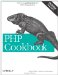# Recipe 9.4. Validating Form Input: Email Addresses

#### 9.4.1. Problem

You want to know whether an email address a user has provided is valid.

#### 9.4.2. Solution

Use the is_valid_email_address( ) function in Example 9-10. It tells you whether an email address is valid according to the rules in RFC 822.

 ` function is_valid_email_address(\$email){ \$qtext = '[^\\x0d\\x22\\x5c\\x80-\\xff]'; \$dtext = '[^\\x0d\\x5b-\\x5d\\x80-\\xff]'; \$atom = '[^\\x00-\\x20\\x22\\x28\\x29\\x2c\\x2e\\x3a-\\x3c'. '\\x3e\\x40\\x5b-\\x5d\\x7f-\\xff]+'; \$quoted_pair = '\\x5c[\\x00-\\x7f]'; \$domain_literal = "\\x5b(\$dtext|\$quoted_pair)*\\x5d"; \$quoted_string = "\\x22(\$qtext|\$quoted_pair)*\\x22"; \$domain_ref = \$atom; \$sub_domain = "(\$domain_ref|\$domain_literal)"; \$word = "(\$atom|\$quoted_string)"; \$domain = "\$sub_domain(\\x2e\$sub_domain)*"; \$local_part = "\$word(\\x2e\$word)*"; \$addr_spec = "\$local_part\\x40\$domain"; return preg_match("!^\$addr_spec\$!", \$email) ? 1 : 0; } if (is_valid_email_address('cal@example.com')) { print 'cal@example.com is a valid e-mail address'; } else { print 'cal@example.com is not a valid e-mail address'; } `

#### 9.4.3. Discussion

RFC 822 defines the standards for a valid email address. The function in Example 9-10, by Cal Henderson, uses the grammar rules laid out in that RFC to build a regular expression. You can read more about how the function is constructed at http://www.iamcal.com/publish/articles/php/parsing_email. Cal has also written a function that validates according to the more complicated rules in RFC 2822. That function is available for download from http://code.iamcal.com/php/rfc822/rfc822.phps.

RFC 822 at http://www.faqs.org/rfcs/rfc822.html, RFC 2822 at http://www.faqs.org/rfcs/rfc2822.html, "Parsing Email Addresses in PHP" by Cal Henderson at http://www.iamcal.com/publish/articles/php/parsing_email, and the functions available for download at http://code.iamcal.com/php/rfc822/.PHP Cookbook: Solutions and Examples for PHP Programmers
ISBN: 0596101015
EAN: 2147483647
Year: 2006
Pages: 445

Similar book on Amazon